# Chapter - Superheterodyne Receiver; PPT; ADC; Semester; Engineering Notes - Computer Science Engineering (CSE)

## Computer Science Engineering (CSE): Chapter - Superheterodyne Receiver; PPT; ADC; Semester; Engineering Notes - Computer Science Engineering (CSE)

The document Chapter - Superheterodyne Receiver; PPT; ADC; Semester; Engineering Notes - Computer Science Engineering (CSE) is a part of Computer Science Engineering (CSE) category.
All you need of Computer Science Engineering (CSE) at this link: Computer Science Engineering (CSE)

Introduction

The slides cover the following topics:

2. Up Conversion

3. Down Conversion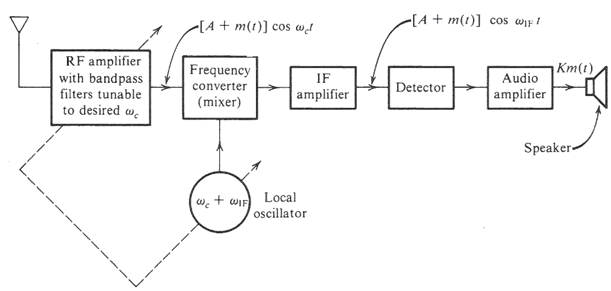Prepared by :  Abdullah Ba-Sulaiman  & Yasser Badahdah, EE370, Sec# 1,   Edited by : Dr. Ali Muqaibel

What is the intermediate frequency fif?

• It is fixed frequency located at 455 kHz

•  The IF filter is band-pass with center frequency of 455 kHz and bandwidth equal to the bandwidth of one AM channel approximately =10 kHz.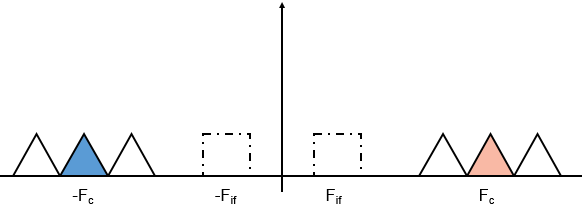Why do we need the IF Stage?

• It  is too difficult to design a tunable and sharp filter. So we design  sharp & fixed filter.

• The channel to be filtered out should first be frequency shifted to the IF frequency by a frequency converter as shown in the super heterodyne Figure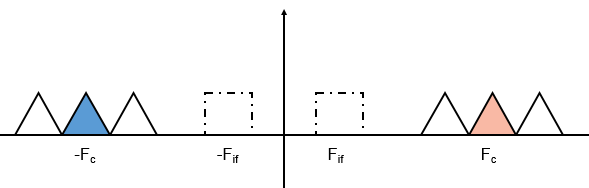Up conversion Fc+Fif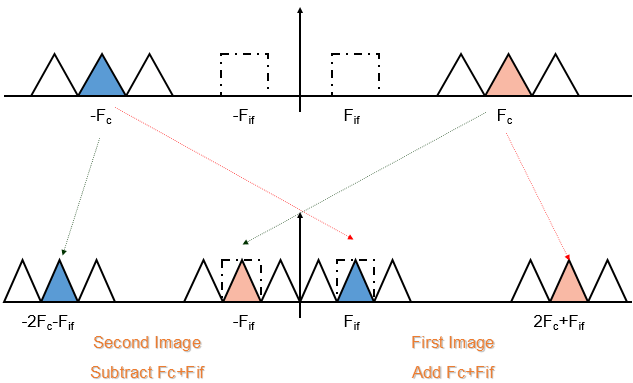Down conversion Fc- Fif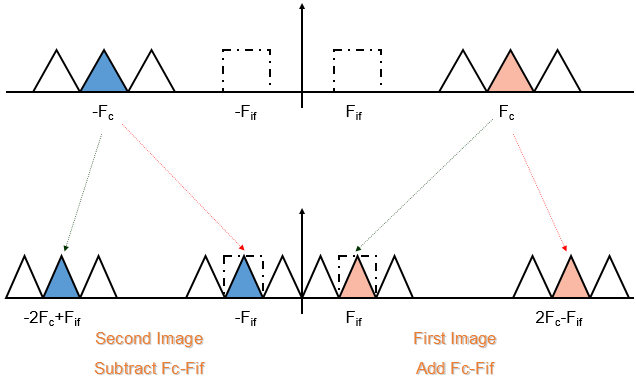Why up conversion is better than down conversion?

The range of radio station on AM is: 550kHz→1600kHz

Up (Fc+Fif): 1005kHz→2055kHz

ratio frequency is 1:2

down (Fc-Fif): 95kHz→1155kHz

ratio frequency is 1:12

We see the ratio frequency in up conversion is smaller than in down conversion which means it is easier to design.

Why we filter at RF stage?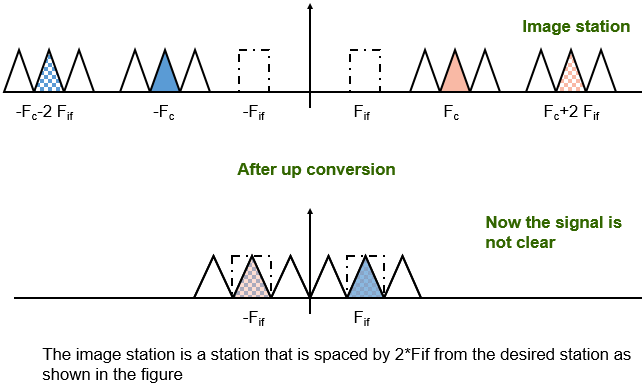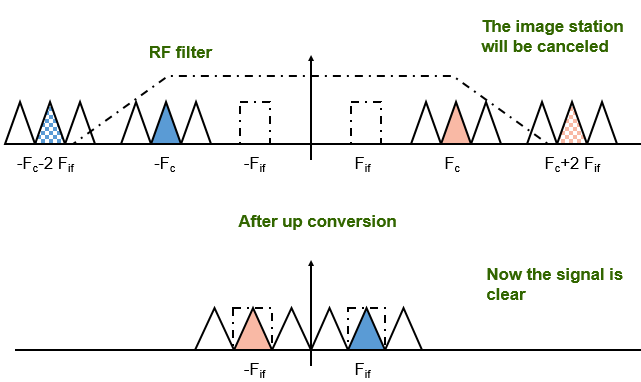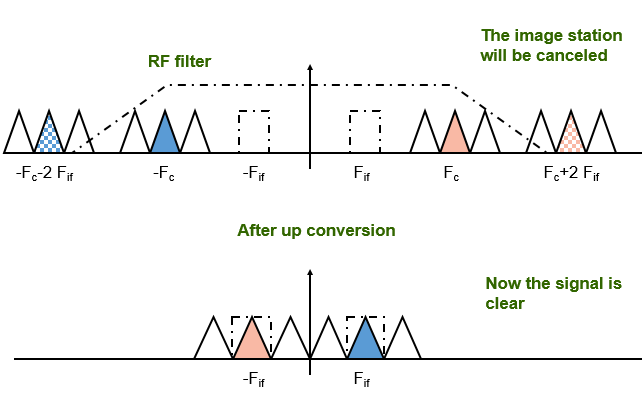The document Chapter - Superheterodyne Receiver; PPT; ADC; Semester; Engineering Notes - Computer Science Engineering (CSE) is a part of Computer Science Engineering (CSE) category.
All you need of Computer Science Engineering (CSE) at this link: Computer Science Engineering (CSE)Use Code STAYHOME200 and get INR 200 additional OFF

Track your progress, build streaks, highlight & save important lessons and more!

,

,

,

,

,

,

,

,

,

,

,

,

,

,

,

,

,

,

,

,

,

;